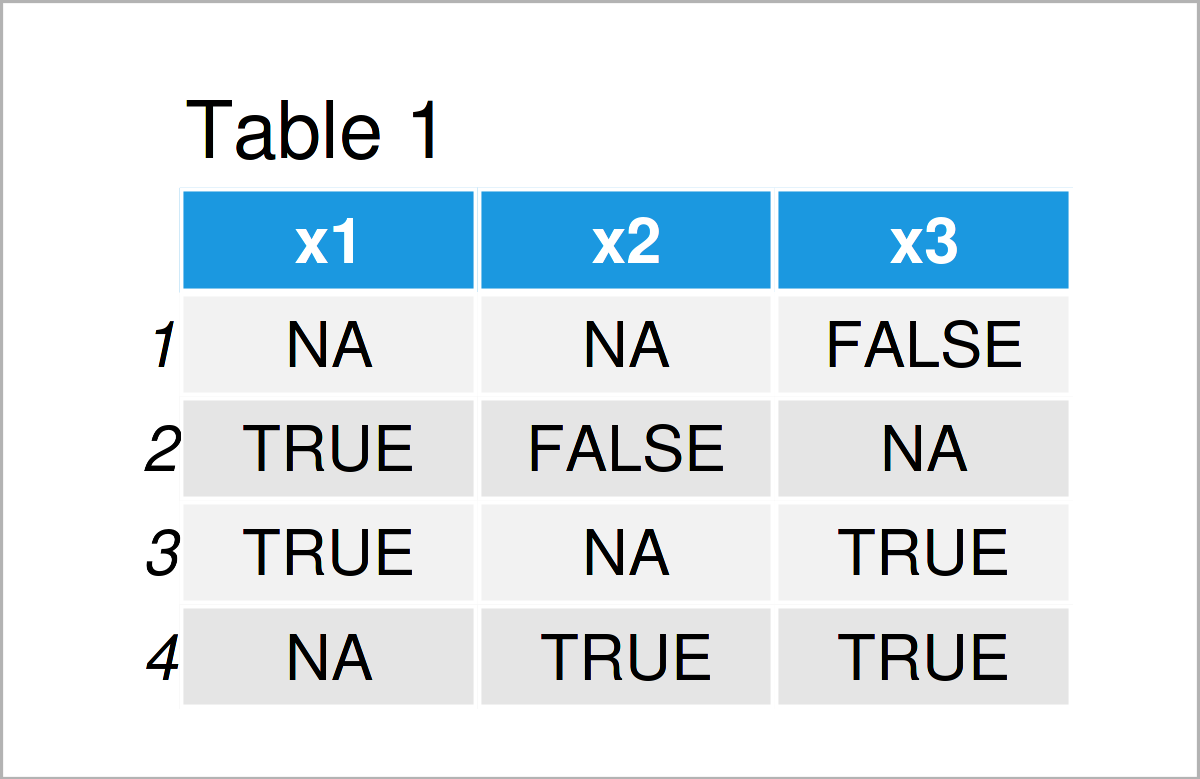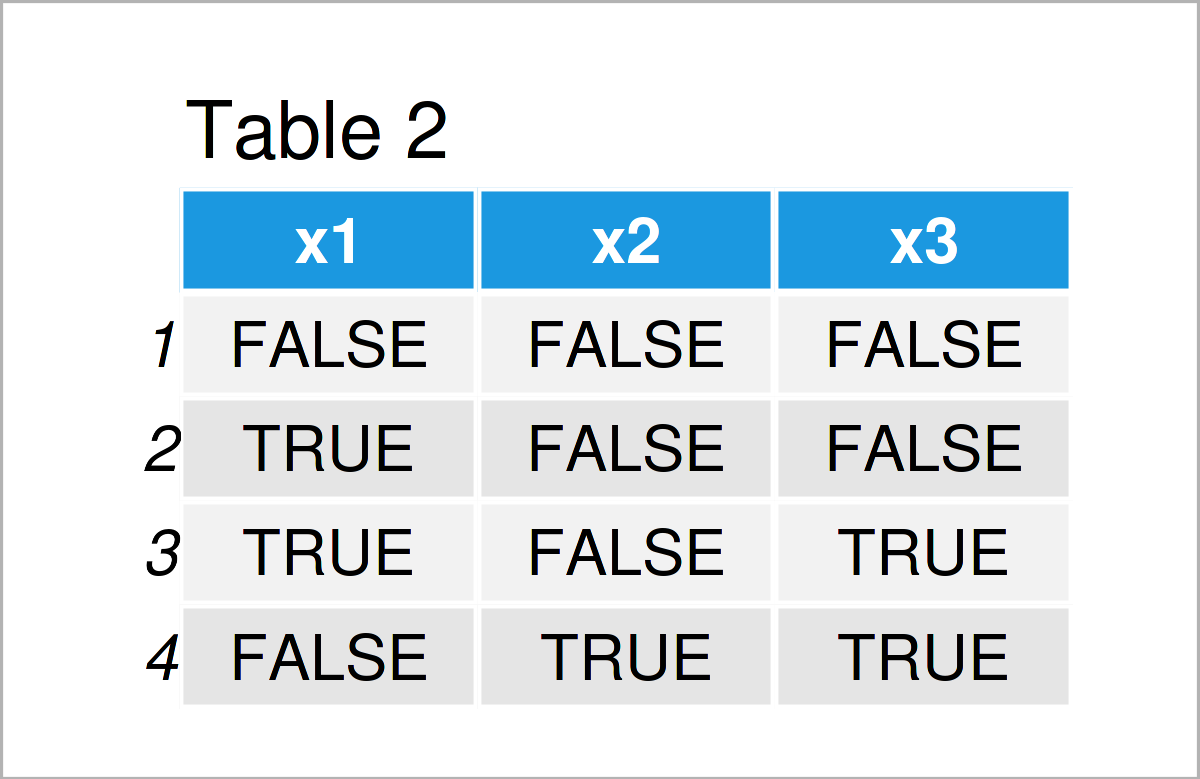# Replace NA by FALSE in R (2 Examples)

This article shows how to exchange NA with FALSE in R programming.

Let’s do this.

## Example Data

First of all, let’s create some example data in R:

```data <- data.frame(x1 = c(NA, TRUE, TRUE, NA),    # Create example data
x2 = c(NA, FALSE, NA, TRUE),
x3 = c(FALSE, NA, TRUE, TRUE))
data                                              # Print example data```Table 1 shows the structure of the example data: It consists of four rows and three logical columns (i.e. TRUE and FALSE). Some of the values in our data are missing (i.e. NA).

## Example 1: Replace NA by FALSE Using Base R

Example 1 illustrates how to substitute all NA values in a data frame by the logical indicator FALSE.

For this task, we can apply the is.na function as shown below:

```data_new1 <- data                                 # Duplicate data
data_new1[is.na(data_new1)] <- FALSE              # Replace NA by FALSE
data_new1                                         # Print updated data```As shown in Table 2, the previous R syntax has created a new data frame called data_new1 where the NA values in all variables have been set to FALSE.

## Example 2: Replace NA by FALSE Using dplyr Package

In Example 2, I’ll show how to use the dplyr package to replace NA by FALSE.

We first need to install and load the dplyr package to R:

```install.packages("dplyr")                         # Install & load dplyr package
library("dplyr")```

Next, we can exchange every not available value by FALSE:

```data_new2 <- data %>%                             # Replace NA by FALSE
replace(is.na(.), FALSE)
data_new2                                         # Print updated data```In Table 3 it is shown that we have created the same output as in Example 1. However, this time we have used the dplyr package instead of Base R.1

## Video & Further Resources

If you need further explanations on the examples of this page, you could watch the following video tutorial on my YouTube channel. I’m explaining the R codes of this tutorial in the video:

In addition, you could have a look at some of the other tutorials on my website.

In this R tutorial you have learned how to replace NA by FALSE in all variables of a data matrix. By the way: A similar syntax might be used to replace only the values in some specific columns. In case you have further questions or comments, let me know in the comments.

Subscribe to the Statistics Globe Newsletter